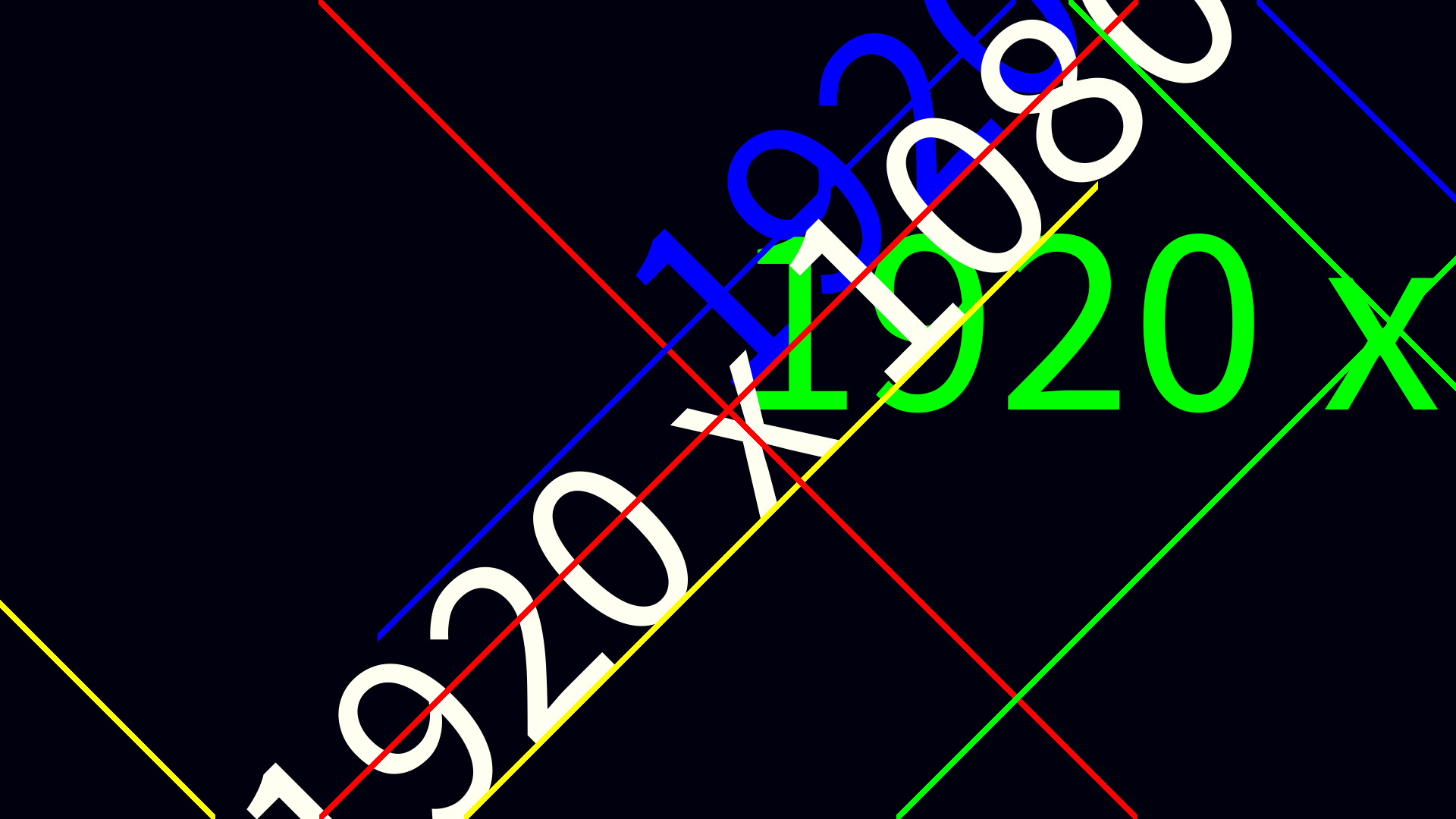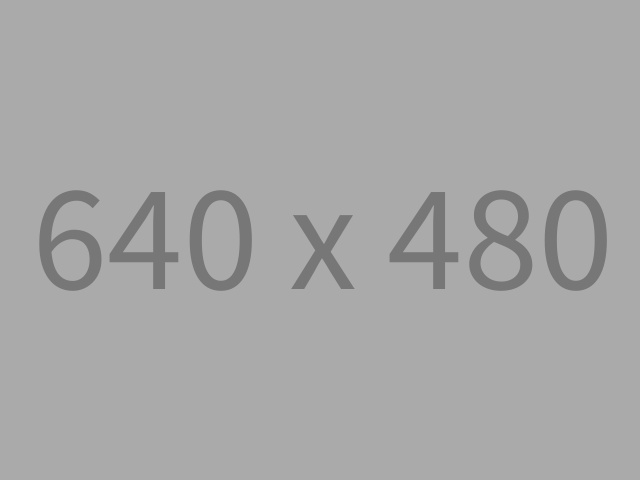### Placeholder Image Generator(Above: An example output with debugging turned on from my placeholder generation service)

For a quite a considerable amount of time now, I've been running my own placeholder image generation service here at starbeamrainbowlabs.com - complete with etag generation and custom colour selection. Although it's somewhat of an Easter Egg, it's not actually that hard to find if you know what you're looking for (hint: I use it on my home page, but you may need to view source to find it).

I decided to post about it now because I've just finished fixing the angle GET parameter - and it was interesting (and hard) enough to warrant a post to remind myself how I did it for future reference. Comment below if you knew about it before this blog post came out!The script itself is split into 3 loose parts:

• The initial settings / argument parsing
• The polyfills and utility functions
• The image generation.

My aim here was to keep the script contained within a single file - so that it fits well in a gist (hence why it currently looks a bit messy!). It's probably best if show the code in full:

(Having trouble viewing code above? Visit it directly here: placeholder.php)

If you append ?help to the url, it will send back a plain text file detailing the different supported parameters and what they do:

(Can't see the above? View it directly here)

Aside from implementing the random value for fg_colour and bg_colour, the angle has been a huge pain. I use GD - a graphics drawing library that's bundled with practically every PHP installation ever - to draw the placeholder image, and when you ask it to draw some rotated text, it decides that it's going to pivot it around the bottom-left corner instead of the centre.

Naturally, this creates some complications. Though some people on the PHP manual page said method (imagettftext) have attempetd to correct for this (exhibits a and b), neither of their solutions work for me. I don't know if it's because their code is just really old (13 and 5 years respectively as of the time of typing) or what.Anyway, I finally decided recently that enough was enough - and set about fixing it myself. Basing my code on the latter of the 2 pre-existing solutions, I tried fixing it - but ended up deleting most of it and starting again. It did give me some idea as to how to solve the problem though - all I needed to do was find the centre of where the text would be drawn when it is both not rotated and rotated and correct for it (these are represented by the green and blue crosses respectively on the image at the top of this post).

PHP does provide a method for calculating the bounding box of some prospective text that you're thinking of drawing via imagettfbbox. Finding the centre of the box though sounded like a horrible maths-y problem that would take me ages to work through on a whiteboard first, but thankfully (after some searching around) Wikipedia had a really neat solution for finding the central point of any set of points.

It calls it the centroid, and claims that the geometric centre of a set of points is simply the average of all the points involved. It just so happens that the geometric centre is precisely what I'm after:

$$C=\frac{a+b+c+d+....}{N}$$

...Where $C$ is the geometric centre of the shape as an $\binom{x}{y}$ vector, $a$, $b$, $c$, $d$, etc. are the input points as vectors, and $N$ is the number of points in question. This was nice and easy to program:

$bbox = imagettfbbox($size, 0, $fontfile,$text);
$orig_centre = [ ($bbox + $bbox +$bbox + $bbox) / 4, ($bbox + $bbox +$bbox + $bbox) / 4 ];$text_width = $bbox -$bbox;
$text_height =$bbox - $bbox;$bbox = imagettfbbox($size,$angle, $fontfile,$text);
$rot_centre = [ ($bbox + $bbox +$bbox + $bbox) / 4, ($bbox + $bbox +$bbox + $bbox) / 4 ]; The odd and even indexes of $bbox there are referring to the $x$ and $y$ co-ordinates of the 4 corners of the bounding boxes - imagettfbbox outputs the co-ordinates in 1 single array for some reason. I also calculate the original width and height of the text - in order to perform an additional corrective translation later.

With these in hand, I could calculate the actual position I need to draw it (indicated by the yellow cross in the image at the top of this post):

$$delta = C_{rotated} - C_{original}$$

.

$$pivot = \frac{pos_x - ( delta_x + \frac{text_width}{2})}{pos_y - delta_y + \frac{text_height}{2}}$$

In short, I calculate the distance between the 2 centre points calculated above, and then find the pivot point by taking this distance plus half the size of the text from the original central position we wanted to draw the text at. Here's that in PHP:

// Calculate the x and y shifting needed
$dx =$rot_centre - $orig_centre;$dy = $rot_centre -$orig_centre;

// New pivot point
$px =$x - ($dx +$text_width/2);
$py =$y - $dy +$text_height/2;

I generated a video of it in action (totally not because I thought it would look cool :P) with a combination of curl, and ffmpeg:

This was really quite easy to generate. In a new folder, I executed the following:

echo {0..360} | xargs -P3 -d' ' -n1 -I{} curl 'https://starbeamrainbowlabs.com/images/placeholder/?width=1920&height=1920&fg_colour=inverse&bg_colour=white&angle={}' -o {}.jpeg
ffmpeg -r 60 -i %d.jpeg -q:v 3 /tmp/text-rotation.webm

The first command downloads all the required frames (3 at a time), and the second stitches them together. The -q:v 3 bit of the ffmpeg command is of note - by default webm videos apparently have a really low quality - this corrects that. Lower is better, apparently - it goes up to about 40 I seem to remember reading somewhere. 3 to 5 is supposed to be the right range to get it to look ok without using too much disk space.That's about everything I wanted to talk about to remind myself about what I did. Let me know if there's anything you're confused about in the comments below, and I'll explain it in more detail.

(Found this interesting? Comment below!)